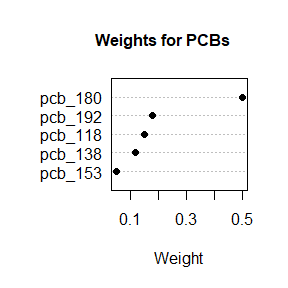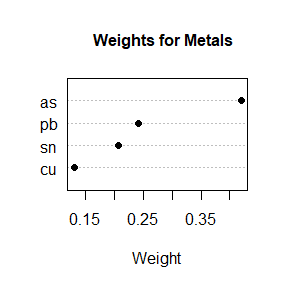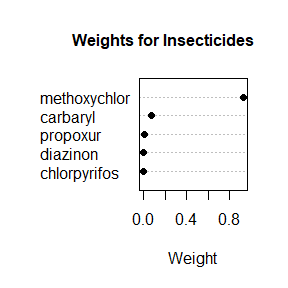# groupWQS Vignette

#### 2020-06-27

library(groupWQS)

# Introduction

Weighted Quantile Sum (WQS) regression (Carrico et al., 2015) was developed to assess the effect of chemical mixtures (usually from the environment) on the risk of an adverse event such as cancer. The method allows one to identify “bad actor” chemicals through non-zero weights where the data are highly correlated and high dimensional (typical in chemical concentration data). Studies have shown it to be more accurate in identifying these chemicals (via sensitivity and specificity) than traditional regression and regularization methods such as LASSO, adaptive LASSO, and elastic net (Czarnota et al., 2015 Cancer Informatics). WQS regression treats all chemicals as being part of one group and having the same direction of association with the outcome. Grouped Weighted Quantile Sum (GWQS) regression (Czarnota and Wheeler, 2016) extends this method to allow one to place chemicals into groups such that different magnitudes and direction of associations can be determined for each pre-defined group of chemicals. For example, some types of chemicals may have a harmful effect (i.e. increase risk of cancer) while others may have a protective effect.

Specifically, GWQS uses data with i=1,…,C components split between j=1,…,K groups. Within each of these K groups, the components are scored into quantiles that can be plausibly combined into an index. The weights $$w_i$$ are constrained to be between 0 and 1 and sum to 1, which in turn addresses potential issues with collinearity and high dimensionality. The general form of GWQS regression can be expressed as:

$\begin{equation} \tag{1} g(\mu)=\beta_{0}+\sum_{j=1}^{K}\left[\beta_{j}\left(\sum_{i=1}^{c_{j}} w_{j i} q_{j i}\right)\right]+z^{T} \phi \end{equation}$

where $$w_{ji}$$ represents the weight for the ith chemical component $$q_{ji}$$, and the summation $$\sum^{c_i}_{i=1}w_{ji}q_{ji}$$ represents a weighted index for the set of $$c_j$$ chemicals of interest within group j. The vector z is a vector of covariates for which to adjust. On the right hand side, g is a monotonic and differentiable link function that relates to the mean $$\mu$$. The link function used depends on the type of outcome (e.g. continuous or binary). For inference, the equation (1) is run for B bootstraps where in each bootstrap sample the significance of the estimated vector of weights is evaluated through the significance (P≤0.05) of $$\hat{\beta}_j$$, the parameter estimate of weighted index j. The estimated index for each of the j chemical groups is obtained by $$GWQS_j = \sum^{c_i}_{i=1}\bar{w_{ji}}q_{ji}$$ where $$\bar{w_{ji}}$$ is the weighted average of the $$\hat{\beta}_j$$ test statistics for component i. Significance of the group effects is assessed using the following model with the test dataset:

$\tag{2} g(\mu)=\beta_{0}+\sum_{j=1}^{K}\left[GWQS_j\right]+z^{T} \phi$

Nonlinear optimization of (1) using the solnp function from R is used to estimate the unknown weights and beta parameters. This function uses an augmented Lagrange multiplier method with a sequential quadratic programming interior algorithm.

The main function of groupWQS is gwqs.fit, which implements GWQS regression. The functions make.X and make.x.s are used to prepare data for regression, where make.X organizes user data into a matrix of desired groups while make.x.s forms a vector detailing the order and size of each group in the exposure matrix. The output of these two functions are used as arguments in the main gwqs.fit function. The model fit object that is returned can be fed into the plot.weights function, which visualizes the weight estimated for individual chemicals in each of the regressed groups. We provide an example analysis below to illustrate the usage of groupWQS.

# Using groupWQS

### Data Preparation

The package groupWQS requires a dataframe where component variables used to construct indices are named. As an example, we will look at simdata:

data(simdata)
#>    pcb_118  pcb_138  pcb_153   pcb_180   pcb_192        as        cu         pb
#> 1 2.360824 2.700214 3.536807 1.3630520  3.568884  4.332258 3.4998226  7.6088824
#> 2 2.788382 1.353622 2.328022 3.3261689 -2.020155 -1.146443 0.8174605 -1.6830696
#> 3 6.246413 3.166612 6.851131 7.2149506 10.504976  5.042383 6.2631718  7.2252882
#> 4 3.642265 2.881070 3.760282 4.3231974  3.368709  2.814906 3.9192663  2.1143266
#> 5 3.481870 3.820329 3.178467 0.9451579  3.786788  6.331203 2.2228106  4.8262313
#> 6 4.088892 4.799032 5.467218 4.2547060  6.078460  2.622098 1.8577607  0.6898549
#>         sn   carbaryl propoxur methoxychlor   diazinon chlorpyrifos Y
#> 1 3.975121  4.7416076 4.203351     4.598234  5.5571762    5.4269398 0
#> 2 1.710898 -0.4558587 2.255870     2.751266  2.6322118   -0.6704027 0
#> 3 4.857086  7.2872371 5.274585     6.739046 12.0197435    8.1984625 0
#> 4 3.798077  0.7455287 3.111616     2.940376  0.6781079    1.5744175 0
#> 5 4.409647  0.6547444 2.309184     3.652926  0.6819997    1.7293713 1
#> 6 4.374542  4.3322814 4.672852     4.023438  6.7486293    5.9936270 1

These data represent chemical concentrations for 14 suspected carcinogens found in the environment, and the Y variable represents our case-control outcomes, 1 for cancer and 0 for cancer-free.

To prepare our data and let gwqs.fit know how many groups we want in our model, as well as which variables belong to which group, we use two functions:

1. make.X

make.X organizes component variables into a matrix suitable for analysis. To specify variable groups, we make a list of string vectors, each vector corresponding to a group and each string corresponding to a variable name. This list is then used as an argument in make.X.

group_list <- list(c("pcb_118", "pcb_138",  "pcb_153", "pcb_180", "pcb_192"),
c("as", "cu", "pb", "sn"),
c("carbaryl", "propoxur", "methoxychlor", "diazinon", "chlorpyrifos"))
X <- make.X(simdata, num.groups = 3, groups = group_list)
#>       pcb_118  pcb_138  pcb_153   pcb_180   pcb_192        as        cu
#> [1,] 2.360824 2.700214 3.536807 1.3630520  3.568884  4.332258 3.4998226
#> [2,] 2.788382 1.353622 2.328022 3.3261689 -2.020155 -1.146443 0.8174605
#> [3,] 6.246413 3.166612 6.851131 7.2149506 10.504976  5.042383 6.2631718
#> [4,] 3.642265 2.881070 3.760282 4.3231974  3.368709  2.814906 3.9192663
#> [5,] 3.481870 3.820329 3.178467 0.9451579  3.786788  6.331203 2.2228106
#> [6,] 4.088892 4.799032 5.467218 4.2547060  6.078460  2.622098 1.8577607
#>              pb       sn   carbaryl propoxur methoxychlor   diazinon
#> [1,]  7.6088824 3.975121  4.7416076 4.203351     4.598234  5.5571762
#> [2,] -1.6830696 1.710898 -0.4558587 2.255870     2.751266  2.6322118
#> [3,]  7.2252882 4.857086  7.2872371 5.274585     6.739046 12.0197435
#> [4,]  2.1143266 3.798077  0.7455287 3.111616     2.940376  0.6781079
#> [5,]  4.8262313 4.409647  0.6547444 2.309184     3.652926  0.6819997
#> [6,]  0.6898549 4.374542  4.3322814 4.672852     4.023438  6.7486293
#>      chlorpyrifos
#> [1,]    5.4269398
#> [2,]   -0.6704027
#> [3,]    8.1984625
#> [4,]    1.5744175
#> [5,]    1.7293713
#> [6,]    5.9936270

We should note that the data in X must be chemical concentrations, which will be converted to quantiles during analysis.

1. make.x.s

make.x.s creates a vector which tells gwqs.fit the size and order of our groups. Conveniently, it uses the same arguments as make.X.

x.s <- make.x.s(simdata, num.groups = 3, groups = group_list)
x.s
#>  5 4 5

With these steps all chemical concentration data have been prepared for analysis. To complete data preparation we define our outcome object

Y <- simdata$Y and split our data into training and validation sets. The training data will be used to estimate the weights in the indices and the validation data will be used to estimate the exposure effects and perform significance testing. Y.train <- Y[1:700] Y.valid <- Y[701:1000] X.train <- X[1:700,] X.valid <- X[701:1000,] ### Model Fitting To fit a model we will use the gwqs.fit function. First we pass the objects defined above (note: If arguments are not supplied for y.train and x.train the y and x data passed will be used for both training and validation). In addition to the x and y parameters there are z and z.train parameters for covariates, but as we are not including covariates in this model these paramters will be left empty. The B parameter tells the function how many bootstrap samples to use in estimation and n.quantiles specifies the number of quantiles to use. The func parameter tells the function which outcome type to fit the model for (continuous and binary outcomes are currently supported). In the example below, five quantiles (quintiles) are used for the chemicals. results <- gwqs.fit(y = Y.valid, y.train = Y.train, x = X.valid, x.train = X.train, x.s = x.s, B=100, n.quantiles = 5, func = "binary") If there are convergence problems after model fitting the tol and delta parameters can be adjusted. A larger tol value will relax the criteria for convergence and a larger delta will increase the step size for the bootstrap procedure; both will speed up convergence. summary(results$fit)$coefficients #> Estimate Std. Error z value Pr(>|z|) #> (Intercept) -0.36296995 0.27747793 -1.3081039 0.1908380843 #> GWQS1 -0.12558969 0.10612361 -1.1834284 0.2366394011 #> GWQS2 0.02733979 0.11027004 0.2479349 0.8041847797 #> GWQS3 0.35752680 0.09285251 3.8504807 0.0001178862 The results above are the regression coefficients for the estimated exposure indices. This is in the validation set. summary(results$fit)$aic #>  405.7135 Next is the AIC for the model we have fit. exp(confint(results$fit))
#> Waiting for profiling to be done...
#>                 2.5 %   97.5 %
#> (Intercept) 0.4016993 1.195476
#> GWQS1       0.7149657 1.084859
#> GWQS2       0.8276495 1.276471
#> GWQS3       1.1949162 1.720857

And finally are the 95% CIs for the odds ratios of the indicies. We can see from the results that of our three groups only the third, GWQS3, is statistically significant at the 0.05 level, and has a 95% CI of (1.2, 1.7) for its odds ratio.

### Examining Individual Component Weights

The weights for individiual chemical components in each group are listed in the object returned by gwqs.fit. To view them graphically in descending order of importance, we can use the plot.weights function. Besides the model fit object, we just need to create a string vector naming our groups. This vector’s order matters - names must match their corresponding index in the group list defined above.

chem_groups <- c("PCBs", "Metals", "Insecticides")
weight.plot(results, chem_groups)Figure 1: Importance plot for chemical concentration weights of GWQS1 indexFigure 2: Importance plot for chemical concentration weights of GWQS2 indexFigure 3: Importance plot for chemical concentration weights of GWQS3 index

Our one significant index was a group of insecticide compounds. Of the five chemicals it appears methoxychlor was mostly responsible for the association GWQS3 had with the outcome variable.